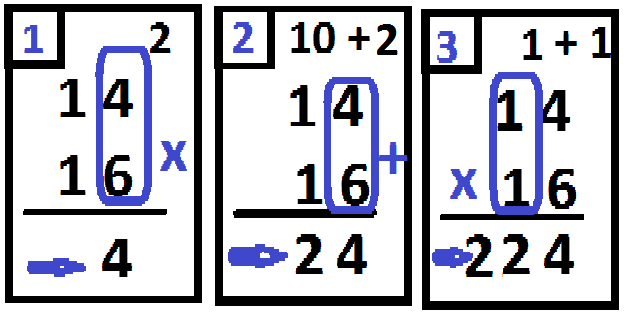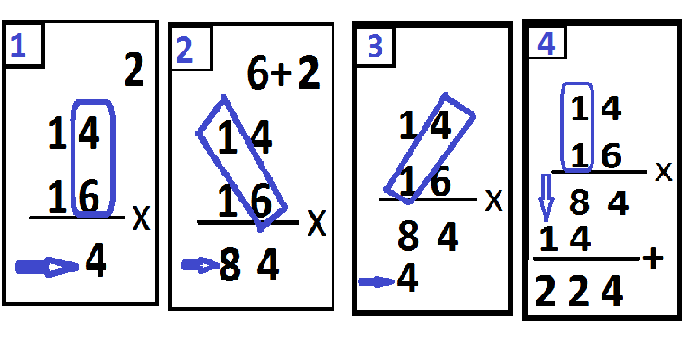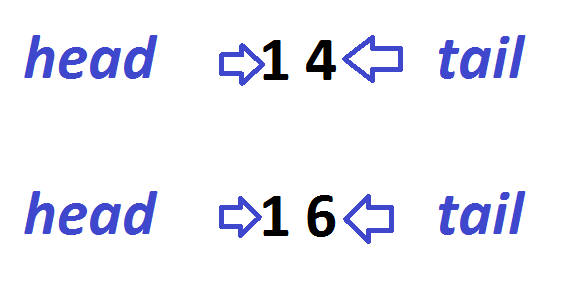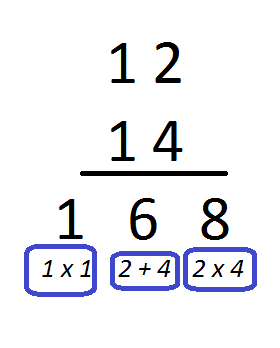# Fast Way to Solving Multiplication of Tens by TensDear all my friends..

People's views of mathematics lessons are many differences. some find it difficult to calculate. Some have difficulty understanding the problem. In fact, mathematics is actually easy. Mathematics is very close to our daily life. We need to change attitudes towards mathematics to be positive and fun.

We need to instill this attitude in children from an early age so that they do not make mathematics a lesson to be avoided. Thus we will grow a young generation who likes mathematics.
Enthusiasm and joy need to be built in finding solutions to math problems. If we solve math problems with enthusiasm and not full of pressure, the results will be optimal. A positive attitude towards learning mathematics will multiply the enthusiasm and energy in solving problems. Children will be able to face contextual questions as challenges, not obstacles. The result will certainly make difficult and complex questions easier.

For example, we can teach you how to multiply numbers with a faster method. If usually multiplication is defined as repeated addition, then of course we have to provide a quick way so that children can solve multiplication more easily.

## Multiplication of tens by tens

For example, the multiplication of the number tens by tens is usually solved in a long or tiered way.

How to do it is done in steps

1. Multiply the first line unit times the second line unit
2. Multiply the units of the second row times the tens of the first row
3. Multiply the second line unit by the first line unit
4. Multiply the tens on the first line and the tens on the second line.

Example:

#### 14 x 16 =...

The multiplication solution can be seen in the following steps:1. Multiply the unit number 4 by the unit 6 = 24, write the number 4, save the number 2
2. Multiply the units digit 6 by the tens 1= 6 add 2 to make 8
3. Multiply the units digit 4 by the tens 1 = 4, write it under the number 8
4. Multiply the tens number 1 by the tens 1 = 1 write in front of 4.
add up the two rows of numbers so that the result is 224.

Well, this time we will learn to multiply 14 and 16 the fast way.
Previously I would like to give a mention

### The unit number we call tail.

Then how to teach the multiplication of tens to our children using the designation of the head and tail.

The steps for doing multiplication are

1. The tail of the tail, if there are dozens, save it
Now let's look at the multiplication of 14 x 16.At number 14.
Number 1 we call the head and 4 is called the tail

At number 16.
Number 1 we call the head and 6 is called the tail

The way to do it is

• Tail times tail, meaning 4 x 6 = 24. Write the number 4 save the number 2
• The tail adds the tail, meaning 4 + 6 = 10, add it to the stored number, which is 2, the result is 12. Write the number 2 and save the number 1.
• Head times head, meaning 1 x 1 = 1 add the number we saved 1 = 2 and then write it in front so that the result will be 224 as can be seen in the following image.We can apply the same method to the multiplication of other tens.
Example:

#### 12 x 14 =...In this way, it will be easier for children to do the multiplication of tens by tens. Children will feel happy to get a faster way.

Thus my writing this time may be useful for all of us.

Best regard from Indonesia.
@rokhani

0
0
0.000